Question

# Optics and Converging & Diverging Lenses 1. What is the relationship between the curvature of a...

Optics and Converging & Diverging Lenses

1. What is the relationship between the curvature of a lens (thick vs thinner converging lenses) and its light-bending power?

2. Focal length: is there a trend in the image distances vis a vis focal lengths as the object distance gets larger? What happens to the thin lens formula when object distance is very large?

3. In the compound lens formula, 1/fcomp = 1/f1 + 1/f2, why does a longer focal lens will not work with the diverging lens to produce an image? What does a negative focal length mean in a diverging lens?

4. what are some advantages and disadvantages of using apertures in optical instrutments such as cameras?

5. What is expected to see on an image when you rotate through all the holes (smallest to largest) in an aperture?

6. In a refracting telescope, what mathematical relationship exists between the distance and the focal lengths of the eyepiece and the objective? How do you estimate the magnifying power of a telescope?

Note: Only the first question will be answered as per guidelines

1.

Thin Lens

The optical axis for a lens is defined as a straight line passing through the center of the lens. A "thin lens" is that which has a very small thickness at the center point of the lens when compared to its focal length. The value of the focal length tells us the "bending power" of the lens. The smaller is the focal length, greater is the bending power. The focal length for a thin lens is governed by the Lensmaker's formula,where

f = focal length, n1,n2 = refractive indices of the medium in which lens is kept and of the material of the lens respectively,

R1,R2 = radius of curvatures of the two lens surfaces

Thick Lens

For a thick lens, the focal length is governed by the Gullstrand’s equation. The focal length is related to the lens thickness at the optical center, focal length of each lens surface, refractive index of the material of the lens.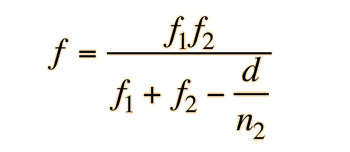where

f1,f2 = focal length of each lens surface

d = thickness of the lens at the optical center

n2 = refractive index of material of which the lens is made.

#### Earn Coins

Coins can be redeemed for fabulous gifts.

Similar Homework Help Questions
• ### Which is true of a compound microscope? Question 1 options: Uses two converging lenses The optics...

Which is true of a compound microscope? Question 1 options: Uses two converging lenses The optics are reversed from those of a refracting telescope The object to be viewed to be enlarged is placed just outside the focal point of the lens All of the above An optical surface is converging if . . . Question 2 options: Parallel incident rays are bent so they intersect after interacting with the surface Parallel incident rays are bent so they intersect before...

• ### The focal lengths of the converging and diverging lenses in the diagram are 9 and -11...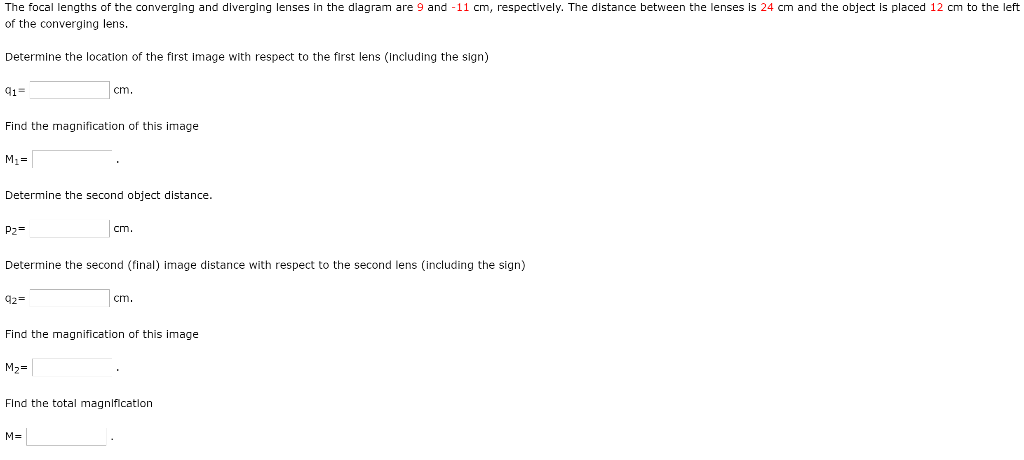The focal lengths of the converging and diverging lenses in the diagram are 9 and -11 cm, respectively. The distance between the lenses is 24 cm and the object is placed 12 cm to the left of the converging lens. Determine the location of the first Image with respect to the first lens (including the sign) 91= cm. Find the magnification of this image Mi= Determine the second object distance, P2= cm. Determine the second (final) image distance with respect...

• ### Optics review D. A converging and diverging lens, each of focal length of magnitude 15.0 cm,...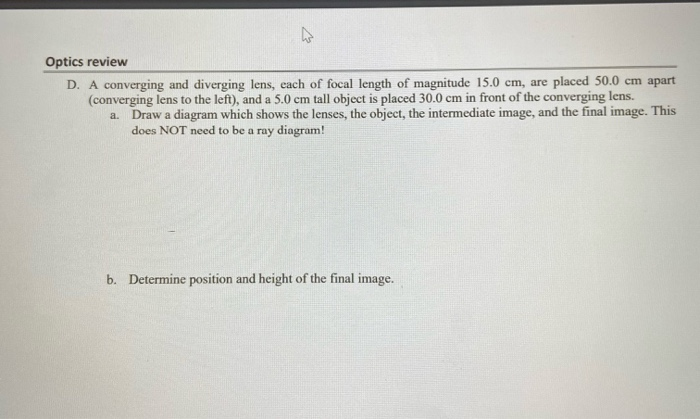Optics review D. A converging and diverging lens, each of focal length of magnitude 15.0 cm, are placed 50.0 cm apart (converging lens to the left), and a 5.0 cm tall object is placed 30.0 cm in front of the converging lens. a. Draw a diagram which shows the lenses, the object, the intermediate image, and the final image. This does NOT need to be a ray diagram! b. Determine position and height of the final image.

• ### converging and diverging lens

Two systems are formed from a converging lens and a diverging lens, as shown in parts a and b of the drawing. (The point labeled "Fconverging" is the focal point ofthe converging lens.) An object is placed inside the focal point of lens 1 at a distance of 6.90 cm to the left of lens 1. The focal lengths of the converging anddiverging lenses are 15.00 and -20.0 cm respectively. The distance between the lenses is 50.0 cm. Determine the...

• ### diverging and converging lenses

A diverging lens with a focal length of -14 cm is placed 10 cm to the right of a converging lens with a focal length of 18 cm. An object is placed 32 cm to the left ofthe converging lens.Where will the final image be located?Where will the image be if the diverging lens is 36 cm from the converging lens?

• ### Please circle final answers The focal lengths of the converging and diverging lenses in the diagram...Please circle final answers The focal lengths of the converging and diverging lenses in the diagram are 7 and -13 cm, respectively. The distance between the lenses is 25 cm and the object is placed 18 cm to the left of the converging lens. Determine the location of the first Image with respect to the first lens (including the sign) 91 cm. Find the magnification of this image M- Determine the second object distance P2 cm. Determine the second (final)...

• ### 2. Two thin lenses, one a converging lens and the other a diverging lens, are arated...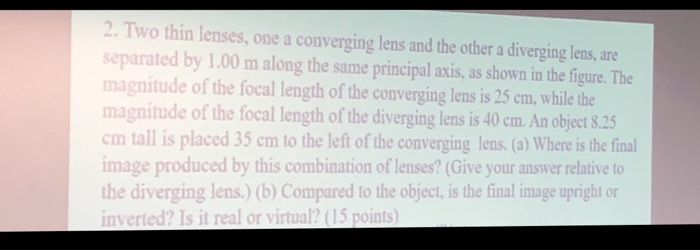2. Two thin lenses, one a converging lens and the other a diverging lens, are arated by 1.00 m along the same principal axis, as shown in the figure. The magnitude of the focal length of the converging lens is 25 cm, while the magnitude of the focal length of the diverging lens is 40 em. An object 8,25 cm tall is placed 35 cm to the left of the converging lens. (a) Where is the final image produced by...

• ### Two systems are formed from a converging lens and a diverging lens, as shown in parts...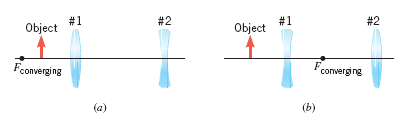Two systems are formed from a converging lens and a diverging lens, as shown in parts a and b of the drawing. (The point labeled "Fconverging" is the focal point of the converging lens.) An object is placed inside the focal point of lens 1 at a distance of 6.4 cm to the left of lens 1. The focal lengths of the converging and diverging lenses are 15.0 and -20.0 cm respectively. The distance between the lenses is 50.0 cm....

• ### The Terrestrial Telescope. The converging objective lens and the diverging eyepiece lens share a common focal...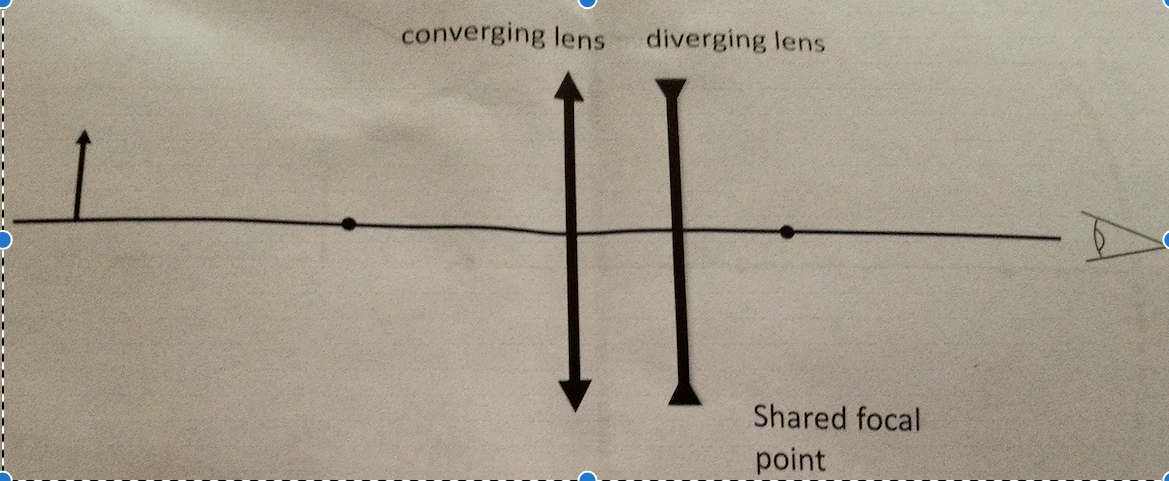The Terrestrial Telescope. The converging objective lens and the diverging eyepiece lens share a common focal point. Note that the other focal point of the diverging lens overlaps the position of the first lens in my particular drawing. This isn’t a necessary condition, just a convenience for the drawing. A) Determine to final image by ray tracing. Trace and label the image of the first lens, then use this image as the object for the second lens. Clearly label the...

• ### ​A converging lens is placed at x 0, a distance d 11.5 cm to the left of a diverging lens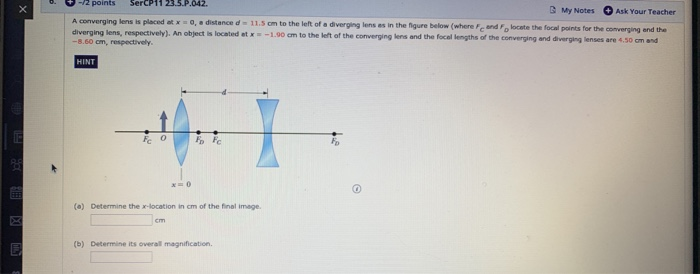A converging lens is placed at x 0, a distance d 11.5 cm to the left of a diverging lens as in the figure below (where F and Fo locate the focal points for the converging and the diverging lens, respectively). An object is located et x-1.90 cm to the left of the converging lens and the focal lengths of the converging and diverging lenses are 4.50 cm and -8.60 cm, respectively. (a) Determine the x-location in cm of the final...